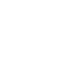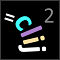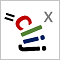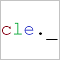# CLIJ2GPU accelerated image processing for everyone

CLIJ2 home

## distanceMatrixToMeshGenerates a mesh from a distance matric and a list of point coordinates.

Takes a pointlist with dimensions nd with n point coordinates in d dimensions and a distance matrix of size nn to draw lines from all points to points if the corresponding pixel in the distance matrix is smaller than a given distance threshold.

Categories: Graphs, Visualisation

Availability: Available in Fiji by activating the update sites clij and clij2. This function is part of clij2_-2.5.0.1.jar.

### Usage in ImageJ macro

``````Ext.CLIJ2_distanceMatrixToMesh(Image pointlist, Image distance_matrix, Image mesh_destination, Number maximum_distance);
``````

### Usage in object oriented programming languages

Java
```// init CLIJ and GPU
import net.haesleinhuepf.clij2.CLIJ2;
import net.haesleinhuepf.clij.clearcl.ClearCLBuffer;
CLIJ2 clij2 = CLIJ2.getInstance();

// get input parameters
ClearCLBuffer pointlist = clij2.push(pointlistImagePlus);
ClearCLBuffer distance_matrix = clij2.push(distance_matrixImagePlus);
mesh_destination = clij2.create(pointlist);
float maximum_distance = 1.0;
```
```// Execute operation on GPU
clij2.distanceMatrixToMesh(pointlist, distance_matrix, mesh_destination, maximum_distance);
```
```// show result
mesh_destinationImagePlus = clij2.pull(mesh_destination);
mesh_destinationImagePlus.show();

// cleanup memory on GPU
clij2.release(pointlist);
clij2.release(distance_matrix);
clij2.release(mesh_destination);
```
Matlab
```% init CLIJ and GPU
clij2 = init_clatlab();

% get input parameters
pointlist = clij2.pushMat(pointlist_matrix);
distance_matrix = clij2.pushMat(distance_matrix_matrix);
mesh_destination = clij2.create(pointlist);
maximum_distance = 1.0;
```
```% Execute operation on GPU
clij2.distanceMatrixToMesh(pointlist, distance_matrix, mesh_destination, maximum_distance);
```
```% show result
mesh_destination = clij2.pullMat(mesh_destination)

% cleanup memory on GPU
clij2.release(pointlist);
clij2.release(distance_matrix);
clij2.release(mesh_destination);
```
Icy JavaScript
```// init CLIJ and GPU
importClass(net.haesleinhuepf.clicy.CLICY);
importClass(Packages.icy.main.Icy);

clij2 = CLICY.getInstance();

// get input parameters
pointlist_sequence = getSequence();
pointlist = clij2.pushSequence(pointlist_sequence);
distance_matrix_sequence = getSequence();
distance_matrix = clij2.pushSequence(distance_matrix_sequence);
mesh_destination = clij2.create(pointlist);
maximum_distance = 1.0;
```
```// Execute operation on GPU
clij2.distanceMatrixToMesh(pointlist, distance_matrix, mesh_destination, maximum_distance);
```
```// show result
mesh_destination_sequence = clij2.pullSequence(mesh_destination)
// cleanup memory on GPU
clij2.release(pointlist);
clij2.release(distance_matrix);
clij2.release(mesh_destination);
```
clEsperanto Python (experimental)
```import pyclesperanto_prototype as cle

cle.distance_matrix_to_mesh(pointlist, distance_matrix, mesh_destination, maximum_distance)

```

Imprint